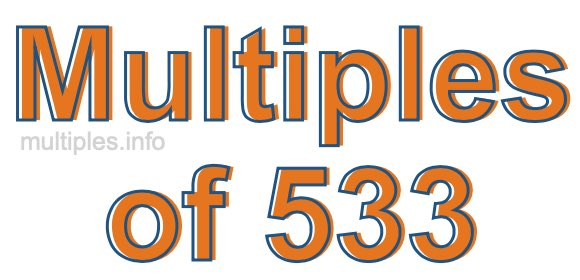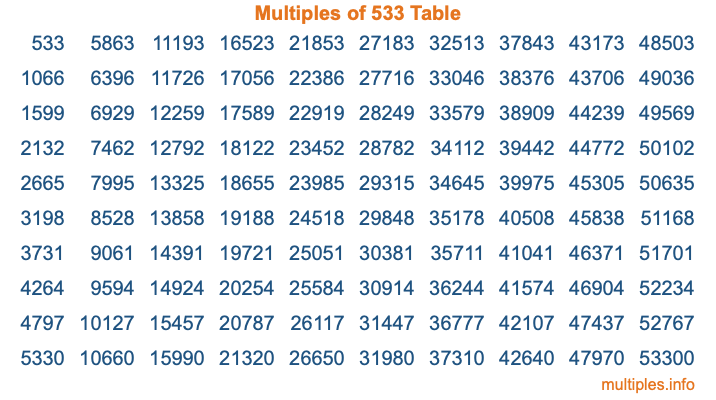Multiples of 533Welcome to the Multiples of 533 page. Here we will first teach you everything you will ever need to know about the multiples of 533, and then give you a study guide summary of everything we taught you to make sure you remember it all. Use this page to look up facts and learn information about the multiples of 533. This page will make you a multiples of five hundred thirty-three expert!

Definition of Multiples of 533
Multiples of 533 are all the numbers that when divided by 533 equal an integer. Each of the multiples of 533 are called a multiple. A multiple of 533 is created by multiplying 533 by an integer.

Therefore, to create a list of multiples of 533, you start with 1 multiplied by 533, then 2 multiplied by 533, then 3 multiplied by 533, and so on for as long as you want. Thus, the list of the first five multiples of 533 is 533, 1066, 1599, 2132, and 2665. To see a larger list of multiples of 533, see the printable image of Multiples of 533 further down on this page. We also have a category where you can choose any nth multiple of 533.

Multiples of 533 Checker
The Multiples of 533 Checker below checks to see if any number of your choice is a multiple of 533. In other words, it checks to see if there is any number (integer) that when multiplied by 533 will equal your number. To do that, we divide your number by 533. If the the quotient is an integer, then your number is a multiple of 533.

Is  a multiple of 533?

Least Common Multiple of 533 and ...
A Least Common Multiple (LCM) is the lowest multiple that two or more numbers have in common. This is also called the smallest common multiple or lowest common multiple and is useful to know when you are adding our subtracting fractions. Enter one or more numbers below (533 is already entered) to find the LCM.

Check out our LCM Calculator if you need more details about the Least Common Multiple or if you need the LCM for different numbers for adding and subtraction fractions.

nth Multiple of 533
As we stated above, 533 is the first multiple of 533, 1066 is the second multiple of 533, 1599 is the third multiple of 533, and so on. Enter a number below to find the nth multiple of 533.

th multiple of 533

Multiples of 533 vs Factors of 533
533 is a multiple of 533 and a factor of 533, but that is where the similarities end. All postive multiples of 533 are 533 or greater than 533. All positive factors of 533 are 533 or less than 533.

Below is the beginning list of multiples of 533 and the factors of 533 so you can compare:

Multiples of 533: 533, 1066, 1599, 2132, 2665, etc.

Factors of 533: 1, 13, 41, 533

As you can see, the multiples of 533 are all the numbers that you can divide by 533 to get a whole number. The factors of 533, on the other hand, are all the whole numbers that you can multiply by another whole number to get 533.

It's also interesting to note that if a number (x) is a factor of 533, then 533 will also be a multiple of that number (x).

Multiples of 533 vs Divisors of 533
The divisors of 533 are all the integers that 533 can be divided by evenly. Below is a list of the divisors of 533.

Divisors of 533: 1, 13, 41, 533

The interesting thing to note here is that if you take any multiple of 533 and divide it by a divisor of 533, you will see that the quotient is an integer.

Multiples of 533 Table
Below is an image of the first 100 multiples of 533 in a table. The table is in chronological order, column by column. The first column has the first ten multiples of 533, the second column has the next ten multiples of 533, and so on.The Multiples of 533 Table is also referred to as the 533 Times Table or Times Table of 533. You are welcome to print out our table for your studies.

Negative Multiples of 533
Although not often discussed or needed in math, it is worth mentioning that you can make a list of negative multiples of 533 by multiplying 533 by -1, then by -2, then by -3, and so on, to get the following list of negative multiples of 533:

-533, -1066, -1599, -2132, -2665, etc.

Multiples of 533 Summary
Below is a summary of important Multiples of 533 facts that we have discussed on this page. To retain the knowledge on this page, we recommend that you read through the summary and explain to yourself or a study partner why they hold true.

There are an infinite number of multiples of 533.

A multiple of 533 divided by 533 will equal a whole number.

533 divided by a factor of 533 equals a divisor of 533.

The nth multiple of 533 is n times 533.

The largest factor of 533 is equal to the first positive multiple of 533.

533 is a multiple of every factor of 533.

533 is a multiple of 533.

A multiple of 533 divided by a divisor of 533 equals an integer.

533 divided by a divisor of 533 equals a factor of 533.

Any integer times 533 will equal a multiple of 533.

Multiples of a Number
Here you can get the multiples of another number, all with the same attention to detail as we did for multiples of 533 on this page.

Multiples of
Multiples of 534
Did you find our page about multiples of five hundred thirty-three educational? Do you want more knowledge? Check out the multiples of the next number on our list!